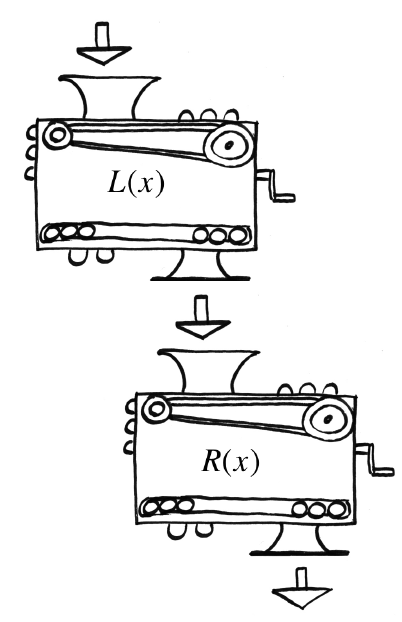### Home > CCA2 > Chapter Ch5 > Lesson 5.1.2 > Problem5-34

5-34.Lacey and Richens each have their own personal function machines. Lacey’s machine, $L(x)$, squares the input and then subtracts one. Richens’s function machine, $R(x)$, adds $2$ to the input and then multiplies the result by three.

1. Write the equations that represent $L(x)\ \text{and}\ R(x)$.

$L(x)=x^2−1$

$R(x)=3(x+2)$

2. Lacey and Richens decide to connect their two machines, so that Lacey’s output becomes Richens’s input. If $3$ is the initial input, what is the eventual output?

$30$

3. What if the order of the machines was changed? Would it change the output? Justify your answer.

Try computing the result for $x=3$ if the order is reversed.